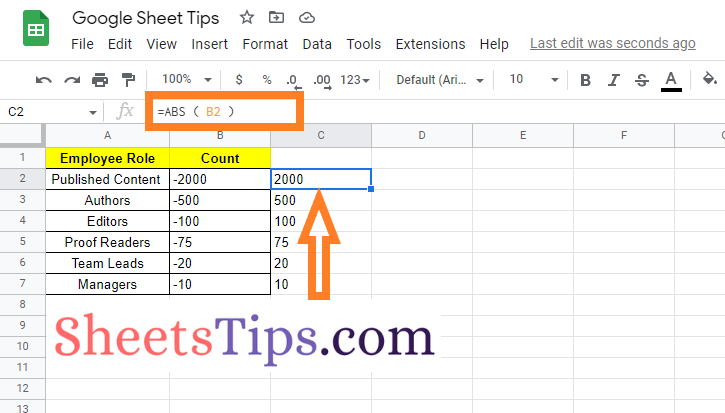# How To Convert Number Signs in Google Sheets? Negative to Positive Conversion

It is no surprise that Google Sheets lets users change the number signs in the available dataset. Spreadsheet users will have various reasons for changing the number signs in the given dataset. The change or reverse of values in the spreadsheet helps to prepare the data quickly without any manual work. And the best part of changing the number signs in Google Sheets is that we have more than one method to change the signs. So, on this page, let us discuss all the methods through which we can change the number signs in the spreadsheet using the Google Sheets Tips. Scroll down to find out more.

## How Do I Convert a Negative Number to a Positive Number in Google Sheets?

There are various methods with the help of which we can convert a negative number to a positive number. Let us discuss each in the below section.

### Changing the Number Signs Using the Find and Replace Method

This is one of the old-style methods that help to change the negative number into a positive one. The steps to change the number sign using Find and Replace are given below:

• 2nd Step: Now click on the “Edit” tab from the menubar and choose “Find and replace” from the drop-down menu.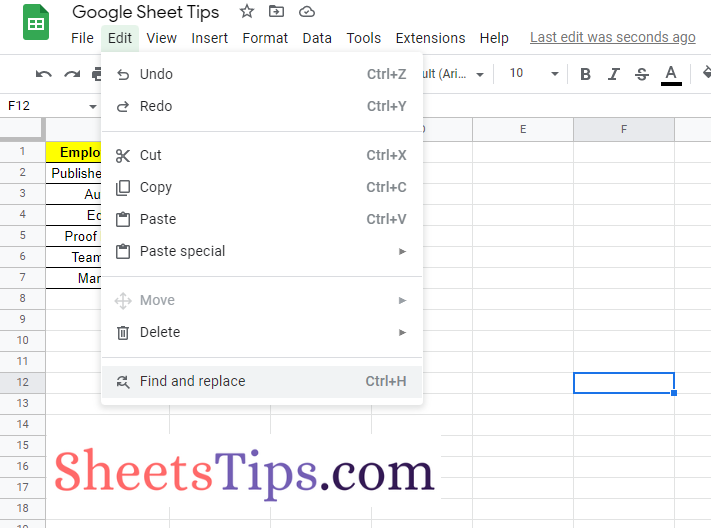• 3rd Step: Here the “Find and replace” drop down will open on the screen. Now in the “Find” text box, enter the minus sign.
• 4th Step: Leave a blank in the “Replace” box.
• 5th Step: Click on the “Replace all” button.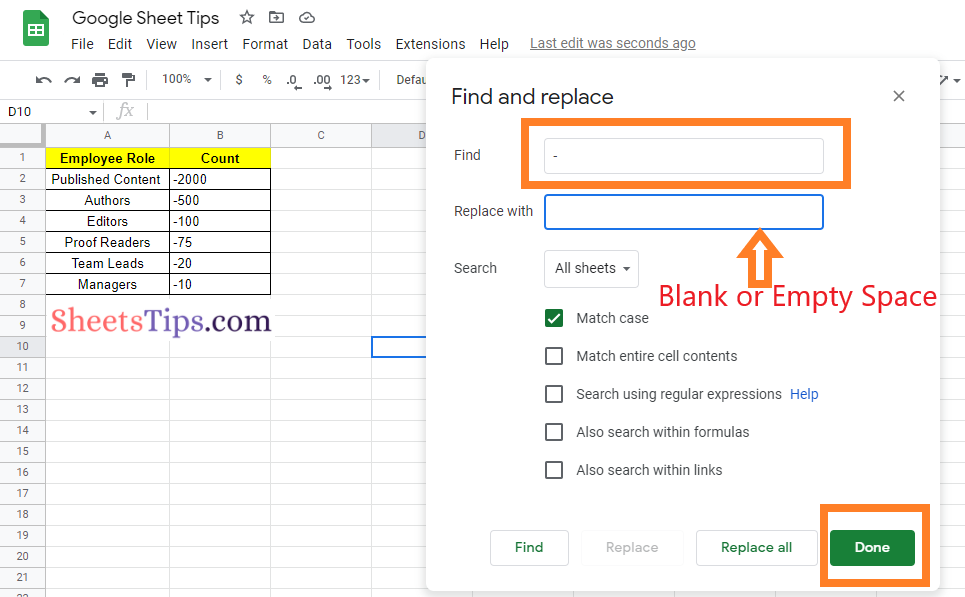• 6th Step: You will see the text being replaced. Now press the “Done” button and you will see the results as shown below.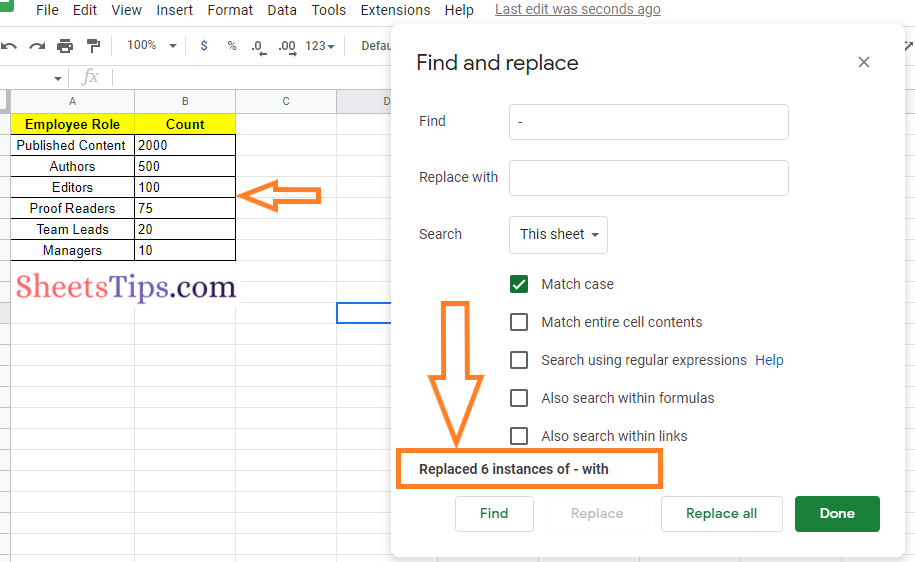### Converting Negative Numbers to Positive Numbers Using the MULTIPLY Function

The multiply function in Google Sheets returns the product of the numbers. Multiplying a negative sign with a negative sign will result in a plus sign. So in our example, we can simply multiply the negative numbers by minus 1, which results in a change of signs but not the numbers. Follow the steps given below to convert a negative number to a positive number using the MULTIPLY function.

• 2nd Step: Now on the homepage, move to the cell where you want to show the results of the converted sign.
• 3rd Step: Enter the formula “=MULTIPLY (A2)“.
• 4th Step: Press the Enter key and you will see the results as shown below.
• 5th Step: Drag the fill handle from the formula applied cell to other parts of the cell to convert an array of negative numbers to positive numbers.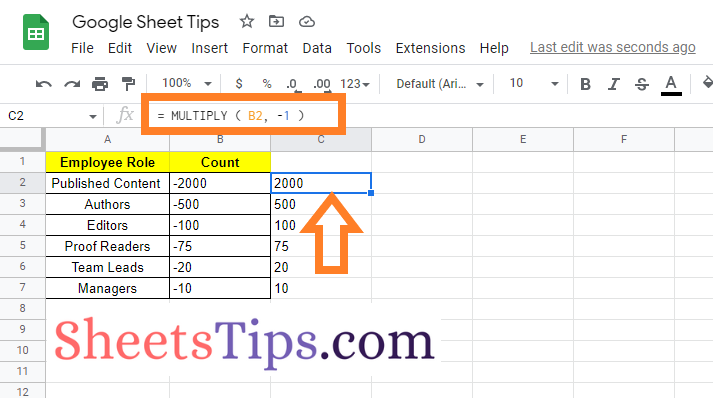### Converting Negative Numbers to Positive Numbers Using the UMINUS Function

The UMINUS function in Google Sheets is a useful operator for reversing the sign of an integer. In fact, you can reverse the sign of numbers in Google Sheets without using this operator function. The steps to using the UMINUS function to change negative numbers to positive numbers are outlined below.

• 1st Step: Open the Google Sheets.
• 2nd Step: On the homepage, move to the cell where you want to convert the negative numbers into positive numbers.
• 3rd Step: Now enter the formula “=UMINUS (A2)“.
• 4th Step: Press the “Return” key and you will find the results as shown in the image below.
• 5th Step: Use the fill handle to apply the same formula to other parts of the cell.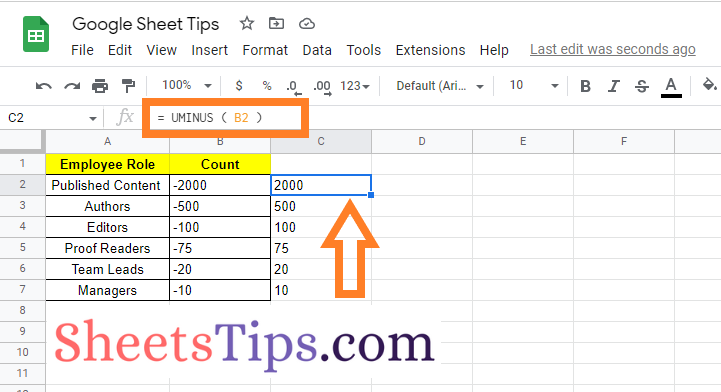### Converting Positive Numbers To Negative Numbers Using The ABS Function

The ABS or Absolute function, in Google Sheets allows users to retrieve absolute values for negative numbers without having to alter cells. The steps to using the ABS function to convert a negative number to a positive number in the spreadsheet are outlined below: